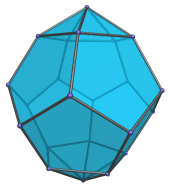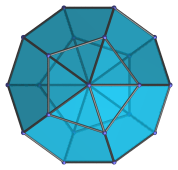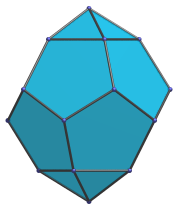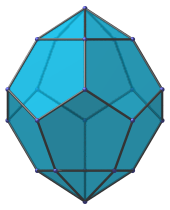# The Parabiaugmented Dodecahedron

The parabiaugmented dodecahedron (J59) is the 59th Johnson solid. It has 22 vertices, 40 edges, and 20 faces (10 pentagons and 10 triangles). It can be constructed augmenting a dodecahedron with two pentagonal pyramids on opposite faces.If one of the pentagonal pyramids is removed, the result is the augmented dodecahedron (J58).

If the two augments lie on pentagons separated by an edge instead of opposite pentagons, the result is the metabiaugmented dodecahedron (J60).

## Projections

Here are some views of the parabiaugmented dodecahedron from various angles:

Projection DescriptionTop view.Side view.Front view.

## Coordinates

The Cartesian coordinates of the parabiaugmented dodecahedron, centered on the origin and with edge length 2, are:

• (0, ±φ2, ±1)
• (±1, 0, ±φ2)
• (±φ2, ±1, 0)
• (±φ, ±φ, ±φ)
• ±(0, (7+φ)/5, (8φ+1)/5)

where φ=(1+√5)/2 is the Golden Ratio.

Last updated 10 Jan 2019.